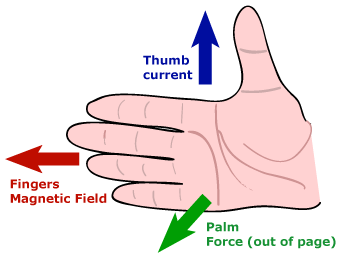## Wednesday, 29 August 2012

### Force on Current-Carrying Conductor in a Magnetic Field

• Interaction of Magnetic Fields:
The Resultant Field with Catapult Effect (a.k.a. Catapult Field)
i.      Just as two bar magnets coming within the magnetic field of each other would experience a force – a push (repulsion) or a pull (attraction) depending on the positions of their magnetic poles:

i.      a current-carrying conductor (which has magnetic field around it) would similarly experience a magnetic force when its magnetic field interacts with the magnetic field of another magnet.

ii.      The interaction produces a resultant magnetic field (a.k.a. catapult field) with magnetic field lines wrapping around the conductor like the stretched catapult producing a catapult effect – that moves the conductor from stronger region of the magnetic field to weaker region.
iii.      Drawing the ‘catapult field’ is one way to determine the direction of movement of the current-carrying conductor in a magnetic field – however, it could be time-consuming. A much simpler method is by the use of Fleming’s Left-hand Rule (or Motor Rule):Alternatively, the Right-hand Slap Rule where the four fingers of the right point in the direction of the magnetic field B and the thumb points in the direction of the current  I, the direction of slapping would be the direction of force F on the conductor.:iv.      Interestingly, the magnetic field of a current-carrying conductor itself would also exert a force on the other magnet which field exerts a force on it - as Hans Christian Oersted discovered in 1820 when the pointer of a compass moved when current was switched on in a nearby circuit – It is this discovery which led to the theory of electromagnetism and its many useful applications.

ii.      Fleming’s Left Hand Rule (Motor Rule)
Please see above for the diagram.

iii.      Factors Affecting Magnitude of the Force on Current-Carrying Conductor in a Magnetic Field:
i.      Magnetic Field Strength (of the other magnet)
ii.      Size of Current (in the conductor causing induced magnetic field)
iii.      Length of Wire or Conductor in the Field
iv.      Angle between Magnetic Field (B) and the Current (I)

iv.      Force between Two Current-Carrying Conductors:i.      The magnetic fields of two current-carrying conductors would interact with each other as would the fields of two permanent magnets.

ii.      If the two conductors are near (but not next) to each other:
i.      They attract each other when the current is flowing in the same direction in both of them;
ii.      They repel each other when the current is flowing in the opposite direction.
The direction of movement of the conductor can be determined by any of the following methods:
a.       Plotting the catapult field
b.      Fleming’s Left-hand Rule
c.       Right-hand Slap Rule

iii.      If the two conductors are next to each other:
i.      They interact to produce a stronger magnetic field when the current is flowing in the same direction in both of them;
ii.      They interact to produce a weaker or neutral field when the current is flowing in the opposite direction.

v.      Turning Effect of a Current-Carrying Coil in a Magnetic Fieldi.      For a coil placed in a magnetic field, when the current flows through it, it flows in opposite directions in opposing arms of the coil – concentric magnetic field lines in opposite directions are therefore produced around both arms.

ii.      These induced magnetic fields interact with the external magnetic field. The resultant magnetic field produces a catapult effect in opposite directions for opposing arms of the coil – forming a couple which produces a turning effect and so the coil rotates.

iii.      The direction of rotation of the coil would reverses when:
i.      The current reverses
ii.      The external magnet poles reverse

The direction of rotation of the coil can be determined by:
1.      Plotting the catapult field; or,
2.      Fleming’s Left-hand Rule; or,
3.      Right-hand Slap Rule

vi.      D.C. Motor – Factors Affecting Speed of Rotationi.      The diagram above shows the construction features of a simple dc motor.

ii.      A dc motor consists of a rectangular coil of wire placed between two permanent magnets (a.k.a. the external magnets).

iii.      Both ends of the coil are soldered to a commutator made up of two semi circular copper rings.

iv.      Two carbon brushes are held against the commutator with slight pressure with the help of springs.

v.      Because of the commutator, current always flow in the same direction for the arm near to the north-pole of the external magnet and in the opposite direction for the other arm of the coil. As a result, the coil rotates in one direction.

vi.      The speed of rotation of the coil can be increased if any of these is increased:
1.      The Magnetic Field Strength (of the external magnet)
2.      The Current flowing in the coil (causing stronger induced magnetic field)
3.      The Number of Turns in the Coil (causing stronger induced magnetic field)
4.      The Area of the Coil
------------------------------------------------------------